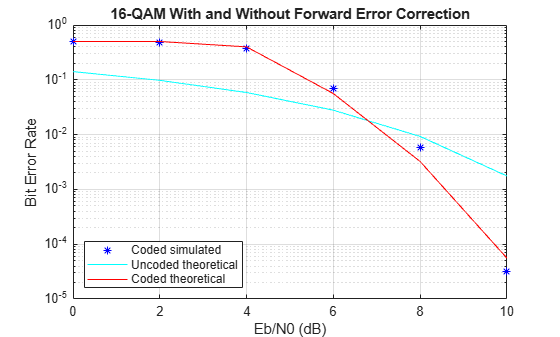# Rate 2/3 Convolutional Code in AWGN

This example generates a bit error rate versus Eb/No curve for a link that uses 16-QAM modulation and a rate 2/3 convolutional code in AWGN.

Set the modulation order, and compute the number of bits per symbol.

```M = 16; k = log2(M);```

Create a trellis for a rate 2/3 convolutional code. Set the traceback and code rate parameters.

```trellis = poly2trellis([5 4],[23 35 0; 0 5 13]); traceBack = 16; codeRate = 2/3;```

Create a convolutional encoder and its equivalent Viterbi decoder.

```convEncoder = comm.ConvolutionalEncoder('TrellisStructure',trellis); vitDecoder = comm.ViterbiDecoder('TrellisStructure',trellis, ... 'InputFormat','Hard','TracebackDepth',traceBack);```

Create an error rate object. Set the receiver delay to twice the traceback depth, which is the delay through the decoder.

`errorRate = comm.ErrorRate('ReceiveDelay',2*traceBack);`

Set the range of Eb/No values to be simulated. Initialize the bit error rate statistics matrix.

```ebnoVec = 0:2:10; errorStats = zeros(length(ebnoVec),3);```

Simulate the link by following these steps:

• Generate binary data.

• Encode the data with a rate 2/3 convolutional code.

• 16-QAM modulate the encoded data, configure bit inputs and unit average power.

• Pass the signal through an AWGN channel.

• 16-QAM demodulate the received signal configure bit outputs and unit average power.

• Decode the demodulated signal by using a Viterbi decoder.

• Collect the error statistics.

```for m = 1:length(ebnoVec) snr = ebnoVec(m) + 10*log10(k*codeRate);```
``` while errorStats(m,2) <= 100 && errorStats(m,3) <= 1e7 dataIn = randi([0 1],10000,1); dataEnc = convEncoder(dataIn); txSig = qammod(dataEnc,M, ... 'InputType','bit','UnitAveragePower',true); rxSig = awgn(txSig,snr); demodSig = qamdemod(rxSig,M, ... 'OutputType','bit','UnitAveragePower',true); dataOut = vitDecoder(demodSig); errorStats(m,:) = errorRate(dataIn,dataOut); end reset(errorRate) end```

Compute the theoretical BER vs. Eb/No curve for the case without forward error correction coding.

`berUncoded = berawgn(ebnoVec','qam',M);`

Plot the BER vs. Eb/No curve for the simulated coded data and the theoretical uncoded data. At higher Eb/No values, the error correcting code provides performance benefits.

```semilogy(ebnoVec,[errorStats(:,1) berUncoded]) grid legend('Coded','Uncoded') xlabel('Eb/No (dB)') ylabel('Bit Error Rate')```